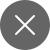# Pandas: 10 minutes 15 basics Part 2

5+

In my previous Pandas tutorial, I discussed in-depth about the basics of Pandas such as importing pandas in python, doing mathematical calculations, accessing data frames, adding labels to the data series, etc to read my previous Pandas tutorial using Python, click ‘here’.

In this tutorial, I will be discussing & showing you the working of how to combine two data frames by using python functions like concat, merge, and join.

We will start by importing the two basic Python libraries:

Create two very small data frames using the DataFrame function:

Read the entire data using the head function, as both the data frame have only 4 rows and 4 columns:

Now, using the function concat() to concatenate the two data frames, in the below code, axis = 0 denotes rows, while axis= 1, denotes columns, sort = True/False parameter is passed to silence a warning which Pandas generate since the version 0.23 was out (current version is 1.0.5):

For axis = 1, sort = True/False:

Using the merge function, how = ‘outer’ is the union of on(AuB), how = ‘inner’ is the intersection of on=’customer’.

And finally concluding with the join function. Join is just like merge, except using one of the values of one of the columns to combine data frames, join uses index labels. Outer, inner, left, right, work the same as merge.

This completes our Pandas tutorial, in our next series of tutorials we will be covering several concepts related to Data Visualization, Machine Learning, etc all of them using Python, till then keep learning, exploring, and upskilling yourself, in these tough times, if you have any queries or you want to learn about R, Tableau, MS-Excel, punch down in the comments section and hit subscribe to receive weekly email updates on news and tutorials related to AI & Data Sciences. To learn NumPy tutorials, click on the below-given links:5+

### 1 Response